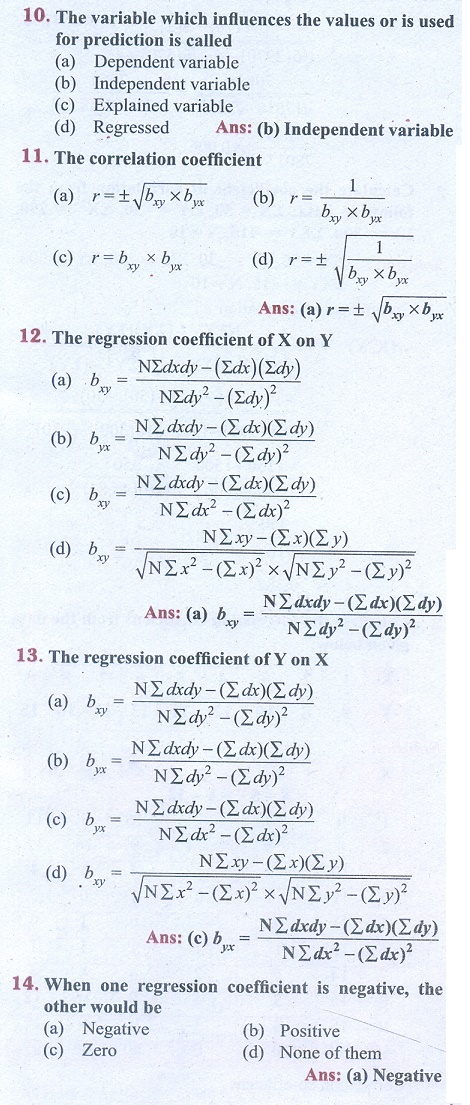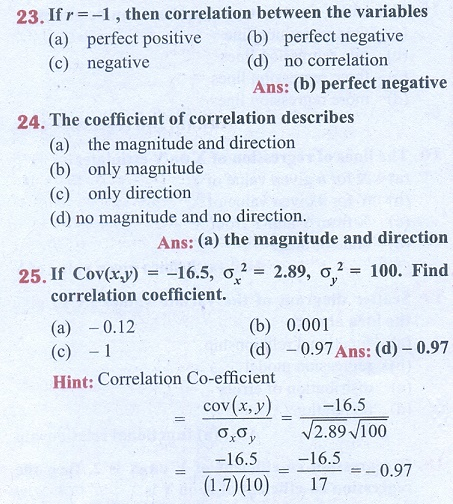Home | | Business Maths 11th std | Exercise 9.3: Choose the correct answer

# Exercise 9.3: Choose the correct answer

Multiple choice questions with answers / choose the correct answer with answers - Business Maths Book back 1 mark questions and answers with solution for Exercise Problems - Statistics: Correlation and Regression analysis

Correlation and Regression analysis - StatisticsTags : Correlation and Regression analysis | Statistics , 11th Business Mathematics and Statistics(EMS) : Chapter 9 : Correlation and Regression analysis
Study Material, Lecturing Notes, Assignment, Reference, Wiki description explanation, brief detail
11th Business Mathematics and Statistics(EMS) : Chapter 9 : Correlation and Regression analysis : Exercise 9.3: Choose the correct answer | Correlation and Regression analysis | Statistics